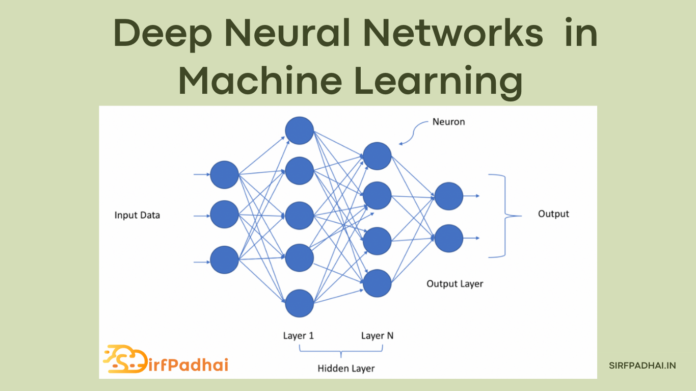# Deep Neural Networks | Principal Components of a Neural Network## What do you understand by deep neural networks?

Neural networks (NN) are powerful statistical learning models that can solve complex classification and regression problems. A neural network is composed of interconnected layers of computation units, mimicking the structures of the brain’s neurons and their connectivity. Each neuron includes an activation function (i.e., a non-linear transformation) to a weighted sum of input values with bias. The predicted output of a neural network is calculated by computing the outputs of each neuron through the network layers in a feed-forward manner. In fact, deep neural networks, which are the backbone of deep learning use a cascade of multiple hidden layers to increase exponentially. the learning capacity of neural networks. Deep neural networks are built using differentiable model fitting, which is an iterative process during which the model trains itself on input data through gradient-based optimization routines, making small adjustments iteratively, with the aim of refining the model until it predicts mostly the right outputs.

### (i) Parameters

It represents the trained weights and biases used by neurons to make their internal calculations.

### (ii) Activations

These represent non-linear functions that add non-linearity to neuron output aimed at indicating, fundamentally, if a neuron should be activated or not.

### (iii) Loss Function

It consists of a math function, which estimates the distance between predicted and actual outcomes. If the DNN’s predictions are perfect, the loss is zero; otherwise, the loss is greater than zero.

### (iv) Regularization

It consists in techniques that penalize the model’s complexity to prevent overfitting such as L1-L2 regularization or dropout.

### (v) Optimizer

It adjusts the parameters of the model iteratively (reducing the objective function), in order to – (a) build the best-fitted model i.e., lowest loss; and (b) keep the model as simple as possible i.e., strong regularization. The most used optimizers are based on gradient descent algorithms.

### (vi) Hyperparameters

These are the model’s parameters that are constant during the training phase and which can be fixed before running the fitting process such as the number of layers or the learning rate.

When training a deep neural network machine learning developers set hyperparameters, and choose loss functions, regularization techniques, and gradient-based optimizers. After training the model, the best-fitted model is evaluated on a testing dataset (which should be different from the training dataset), using error rate and accuracy measures. The occurrence of errors in the training program of a DNN often translates into poor model performance. Therefore, it is important to ensure that DNN program implementations are bug-free. Given the large size of the testing space of a DNN, systematic debugging and testing techniques are required to assist developers in error detection and correction activities.